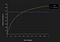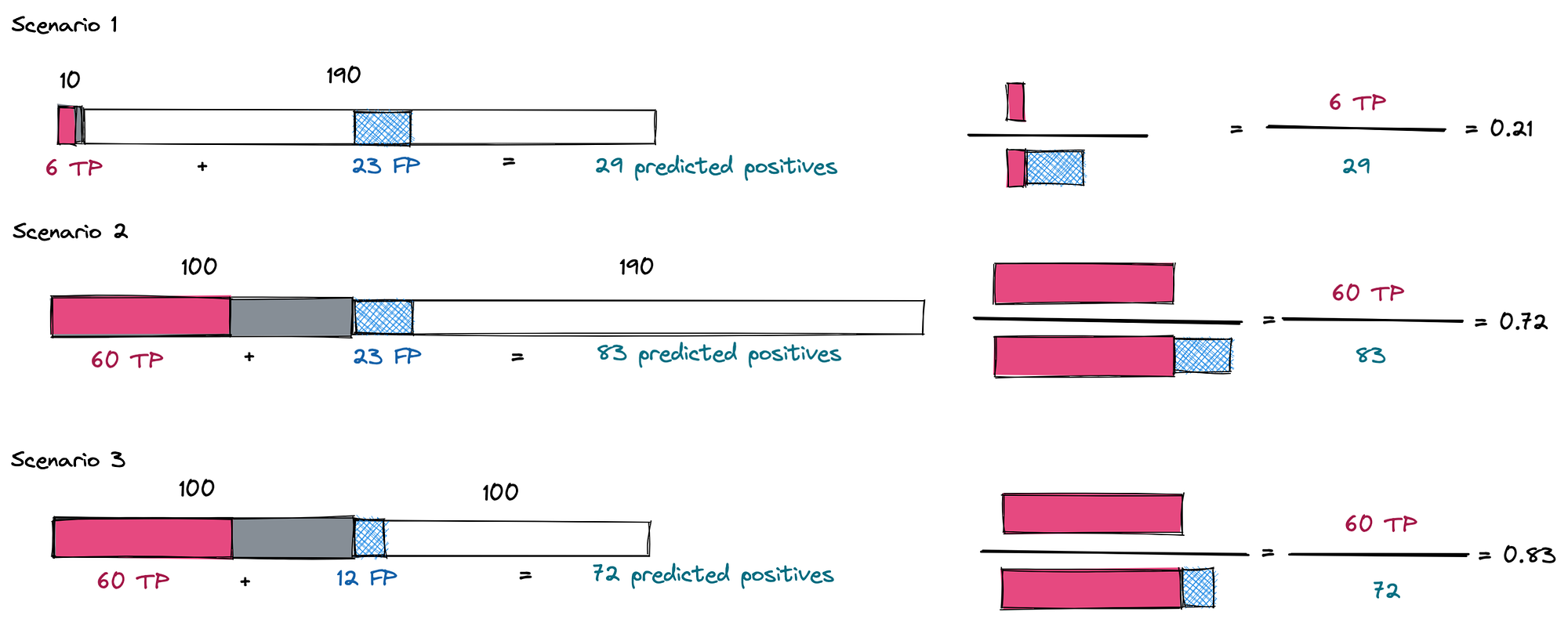# Taking a close look at Precision for classification tasks

At GumGum, we train machine learning models on unstructured data, like text and images. An integral part of model development is model evaluation. Especially for classification tasks, there are several metrics out there. Most known are accuracy, precision, recall, the F-beta score, and the ROC AUC. Each of those gives a slightly different picture of the model’s performance. With this series, I want to give those metrics a fresh look and dive into what each of them can do for you, and, even more importantly, what they cannot do for you.

# Blogs in this Series:

1. Taking a fresh look at metrics for Classification Tasks at GumGum — Introduction
2. Taking a look at Accuracy, Precision, and Recall for Classification tasks
3. Taking a close look at Precision for classification tasks
4. ….

# Overview

In my recent blog post, I talked about accuracy, precision, and recall, and compared them against each other. I mentioned that precision has one property that can lead to wrong conclusions if the Data Scientist is unaware of it. The property I am going to focus on today is precision’s dependence on the class prevalence in the dataset. I am going to look at why this is true and how to work with it to avoid mistakes during model training and model selection, and also how to get the most out of precision.

Please see the previous blog post, if you need a refresher on GumGum’s Brand-Safety classification, which I’ll be using as an example for an imbalanced classification problem with 2 classes. We have one minority class, referred to as “unsafe” and one majority class, referred to as “safe”. The “unsafe” class is the positive class in this example, as this is the class of interest for GumGum.

# Definition: Precision

precision
= # correctly predicted positives / # of predicted positives
= TP / (TP + FP)

# Precision is dependent on the class prevalence

Precision is dependent on the “unsafe”/”safe” balance in the dataset. The more “unsafe” examples there are, the higher precision is; the fewer “unsafe” examples there are, the lower precision is. Intuitively this makes sense because if there are fewer negative examples to be confused with positive examples, precision will be higher than if we had more negative examples than positives. I will go into more detail about this below.

# Deep dive into precision

As mentioned above, the precision score of a model on a dataset is not only dependent on the model’s capability, but also on the class distribution in our dataset. In the image below, I simulated different class imbalances for the “unsafe” class using bootstrapping and calculated precision and recall for a mock-classifier.Precision and Recall plotted against the percentage of the “unsafe” class in the dataset. Created by Sanja Widmer

The below image visualizes the differences between recall and precision. Sensitivity is a synonym for recall, and PPV (positive predictive value) is a synonym for precision.

While specificity and sensitivity are only concerned with their respective class, the PPV and NPV use information from the positive class as well as the negative class.

An increase in the prevalence of the positive class does not affect recall/sensitivity, as both the numerator as well as denominator increase in the same proportions. E.g. if I get 50 out of 100 examples right, this is the same as getting 500 out of 1000 examples right.

However, this is not the case for precision. Precision/PPV’s denominator changes differently than its numerator in the case of a change in the class prevalence. This can be visualized by plugging the areas under the curves of the below visualization into the equations for recall and precision.

The FP in the above visualization is represented by the blue area to the right of the cut-off-2. The TP are the red area to the right of the cutoff-2. With a prevalence increase, the red area to the right of the cut-off will increase, however, the blue area to the right of the cut-off will stay the same. Only if we reduced the blue area, would the blue area to the right of the cut-off become less.

I want to go through 3 examples to better showcase how a shift in class prevalence affects precision.

Let’s say our model:

• classifies 12% of actual negatives as FP (this is the false positive rate and it is model inherent).
• has a recall of 60%.

For the visualizations, I’ll use the following legend.Visualizing precision calculation for different dataset sizes. Created by Sanja Widmer, using Excalidraw

Scenario 1: We have a strongly imbalanced data problem with only 10 positive examples and 190 negative examples (200 examples in total and 5% positive examples). Therefore we will have 190*0.12 = 22.8 FP ~ 23 FP. Also, with a 60% recall we get 0.6*10 = 6 TP.
This means we will have 23 FP + 6 TP = 29 predicted positives.
With this, precision = 6/(23 + 6) = 0.21

Scenario 2: Let’s say we increase the number of positive examples in our dataset to 100 positive examples and keep the negative examples the same. Since we kept the number of negative examples the same, our FP will stay the same. But since we increased the total number of available positives, the number of TP increases to 0.6 *100 = 60 TP.
Therefore we will have 23 FP + 60 TP = 83 predicted positives instead of the previous 29 predicted positives.
With this, precision = 60/(23 + 60) = 0.72.
This is a significant increase in precision, and we only added a few more positive examples!

Scenario 3: Let’s say we keep the overall number of examples in our dataset at 200 and set the number of positives to 100 positive examples and reduce the number of negative examples to 100 as well. Now our data is balanced. Now the number of FP will reduce, it will go down to 100 * 0.12 = 12 FP. The number of TP will be 60.
This then yields 12FP + 60 TP = 72 predicted positives.
Therefore, precision = 60/(12 + 60) = 0.83

→ Drawback 2) We cannot compare the precision score of several models on several datasets with different class prevalences. Therefore, if we decide to collect more data to balance our train and test dataset, an increase in the test data performance in precision cannot necessarily be attributed to a better-performing model. The changed class prevalence in the test data will show an effect.

# Now the big question is: How to use precision properly?

Precision can be very useful during model development, as well as for production monitoring.

For model training, precision is especially useful in conjunction with the negative predictive value (NPV). Just as a reminder, NPV is the number of correct negative predictions out of all the predicted negatives. In the below visualization, we can see a 2-dimensional view of two different models on a given dataset. The dotted area represents the view of one model and the filled areas represent the view of another model. The model with the dotted-area view is better able to differentiate between the positive and the negative class. The overlap between the two areas is lower for the dotted areas than for the filled areas. Since the precision/PPV and NPV use the overlap between the positive and negative class in a model’s predictions. Both precision/PPV and NPV will go down if overlap increases. If overlap decreases, both will go up. Therefore, low precision and low NPV are an indication that the model underfits on the given dataset for this specific class. With this, precision helps us identify the better-performing model.

For production monitoring, precision is most useful if the classification problem requires tight monitoring of FP on the actual number of positive examples in production. Those are classification scenarios where many false positives are detrimental for the business. For instance, let’s say we have a classifier identifying “cat” vs. “no-cat” in production, and GumGum’s advertisers want to display cat food advertisement only on “cat” pages. Then a FP would be detrimental, as the advertiser would pay for ad placements on a non-cat page! Precision will let us know in one number, how we are fairing on that end. Because precision is dependent on the class prevalence, it is supreme to FPR in that case, because we would have to take the FPR does not take the class distribution in our production dataset into consideration.
In Brand Safety, false negatives are detrimental. Therefore, for brand safety, monitoring precision is not as crucial as monitoring recall, it still does give us a good picture of how well the model is able to differentiate positive and negative examples in the production environment. We also monitor the FPR to make sure that we are not filtering too much of our traffic incorrectly. (If you are not sure what Brand-Safety classification is, please check out the first blog post in this series for a quick reminder.)

# Summary

In this blog post, I focused on precision, especially on one property of precision: It is dependent on the class distribution of the dataset and should be used with care. I highlighted the instances where precision is the most useful, and that it should not be used across datasets with different class prevalences.

In the upcoming blog posts, I want to discuss what metrics we can use to complement precision and recall in model selection, and compare different models against each other on the same dataset or even consecutive datasets. We will discuss the harmonic mean between precision and recall, the F1, as well as the weighted harmonic mean between precision and recall, F0.5 and F2. Those especially will help solve the dilemma on how to select a model using only precision and recall. We will also look at the ROC AUC as a way to measure the performance of the model as a whole, independent of the threshold.

Stay tuned :)

We’re always looking for new talent! View jobs.